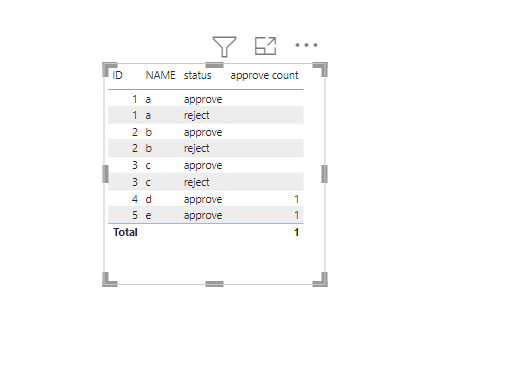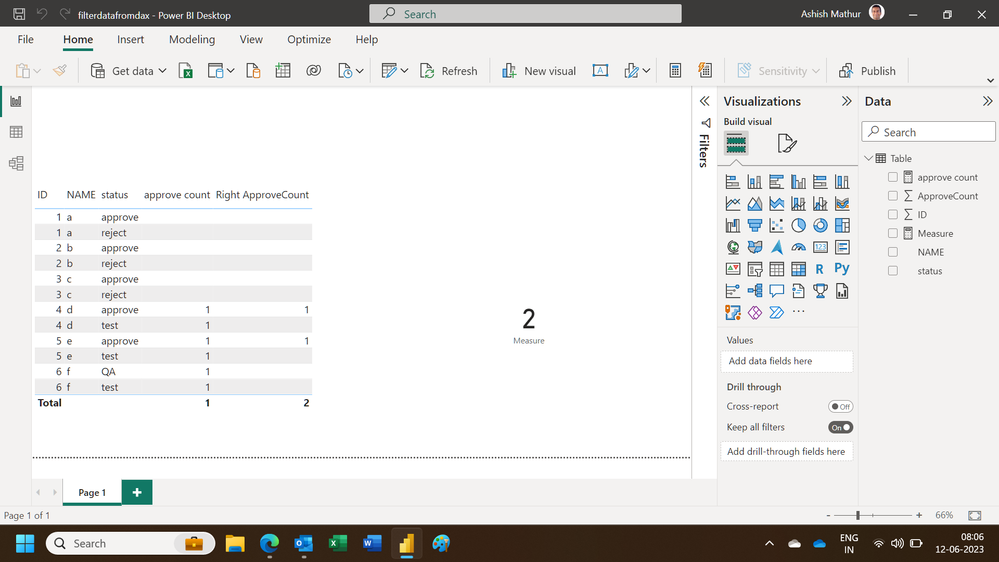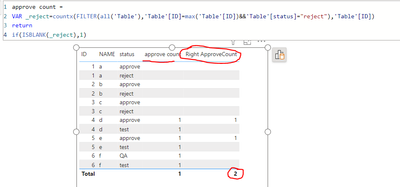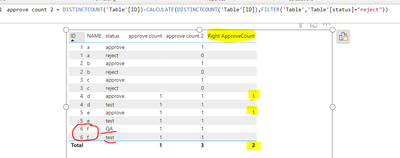cancel
Showing results for
Did you mean:Helper III

## How to filter data from Dax

I have a report like under the screenshot, ID will have a different status,

Want to create a measure to count ID that not including a "Reject" status record,

The highlighted column is what I need, but from Dax, I don't know how to add conditions,2 ACCEPTED SOLUTIONSSuper User

maybe you can try this

``````approve count =
VAR _reject=countx(FILTER(all('Table'),'Table'[ID]=max('Table'[ID])&&'Table'[status]="reject"),'Table'[ID])
return if(ISBLANK(_reject),1)``````pls see the attachment below

Proud to be a Super User!Super User

Hi,

This measure works

``Measure = COUNTROWS(FILTER(VALUES('Table'[ID]),CALCULATE(COUNTROWS('Table'),'Table'[status]="approve")>=1&&CALCULATE(COUNTROWS('Table'),'Table'[status]="reject")=0))``

Hope this helps.Regards,
Ashish Mathur
http://www.ashishmathur.com
8 REPLIES 8Super User

maybe you can try this

``````approve count =
VAR _reject=countx(FILTER(all('Table'),'Table'[ID]=max('Table'[ID])&&'Table'[status]="reject"),'Table'[ID])
return if(ISBLANK(_reject),1)``````pls see the attachment below

Proud to be a Super User!Helper III

@ryan_mayu Hi Ryan, Thank you for your help. But I think I give a wrong example, make you misunderstand, I need count of "Approve" status without Reject ID, for example, I need under result, Do you have any idea? Thank you very muchSuper User

pls try this

``Measure = DISTINCTCOUNT('Table'[ID])-CALCULATE(DISTINCTCOUNT('Table'[ID]),FILTER('Table','Table'[status]="reject"))``pls see the attachmetn below

Proud to be a Super User!Helper III

Thank you for your quick help, this measure does not work for my scenario, in real data sources, it also exists ID not Reject and also not Approve, for example my screenshot ID 6, I need create measure like under hightlight column, just count ID not exist Reject status and when it is apprve. @ryan_mayuSuper User

``Measure =CALCULATE(DISTINCTCOUNT('Table'[ID]),FILTER('Table','Table'[status]="approve"))-CALCULATE(DISTINCTCOUNT('Table'[ID]),FILTER('Table','Table'[status]="reject"))``

Proud to be a Super User!Super User

Hi,

Regards,
Ashish Mathur
http://www.ashishmathur.comHelper IIISuper User

Hi,

This measure works

``Measure = COUNTROWS(FILTER(VALUES('Table'[ID]),CALCULATE(COUNTROWS('Table'),'Table'[status]="approve")>=1&&CALCULATE(COUNTROWS('Table'),'Table'[status]="reject")=0))``

Hope this helps.Regards,
Ashish Mathur
http://www.ashishmathur.com